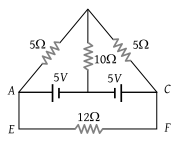In the circuit of adjoining figure the current through 12 Ω resister will be(1) 1 A

(2) $\frac{1}{5}A$

(3) $\frac{2}{5}A$

(4) 0 A

(4) Let the current in 12 Ω resistance is i

Applying loop theorem in closed mesh AEFCA

12i = – E + E = 0

i = 0

Difficulty Level:

• 17%
• 21%
• 5%
• 59%